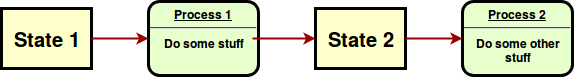# State is processed, not mutated# 進化

## Evolutionary algorithms evolve codified solutions

``````sub random-chromosome( UInt \$length --> List(Seq) )
is export {
return Bool.pick() xx \$length;
}
``````

# ... in populations ...

``````sub initialize( UInt :\$size,
UInt :\$genome-length --> Array ) is export {
my @initial-population;
for 1..\$size -> \$p {
@initial-population.push:
random-chromosome( \$genome-length );
}
return @initial-population;
}``````

## ... through evaluation...

``````multi sub evaluate( :@population,
:%fitness-of,
:\$evaluator --> Mix ) is export {
my MixHash \$pop-bag;
for @population -> \$p {
if  ! %fitness-of{\$p}.defined {
%fitness-of{\$p} = \$evaluator( \$p );
}
\$pop-bag{\$p} = %fitness-of{\$p};
}
return \$pop-bag.Mix;
}``````

## ... to select the best

``````  sub get-pool-roulette-wheel( Mix \$population,
UInt \$need = \$population.elems ) is export {
return \$population.roll: \$need;
}``````

# ... and interchange bits ...

``````    my \$length = @chromosome1.elems;
my \$xover1 = (^(\$length-2)).pick;
my \$xover2 = (\$xover1^..^\$length).pick;
my @x-chromosome = @chromosome2;
my @þone = \$xover1..\$xover2; # crosover zone
@chromosome2[@þone] = @chromosome1[@þone];
@chromosome1[@þone] = @x-chromosome[@þone];
return [@chromosome1,@chromosome2];``````

# ... which mutate ...

``````sub mutation ( @chromosome is copy --> List ) is export {
my \$pick = (^@chromosome.elems).pick;
@chromosome[ \$pick ] = !@chromosome[ \$pick ];
return @chromosome;
}``````

# Perl 6 offers channel based concurrency

``````my Channel \$c .= new;
my Channel \$c2 = \$c.Supply.batch( elems => 2).Channel;
my Channel \$output .= new;
my \$count = 0;
\$c.send(1) for ^2;``````
``````my \$more-work = start react whenever \$c2 -> @item {
if ( \$count++ < 32 ) {
\$c.send( @item);
my \$sum = sum @item;
\$c.send( \$sum );
\$output.send( \$sum );
} else {
\$c.close;
}
}
await \$more-work;
``````

# Connecting to channel evaluation

`````` my @evaluation = ( start react whenever \$raw -> \$one {
my \$with-fitness = \$one => max-ones(\$one);
\$output.send( \$with-fitness );
\$evaluated.send( \$with-fitness); # Check for solution and stuff
``````

``````    my \$selection = ( start react whenever \$channel-three -> @tournament {
my @ranked = @tournament.sort( { .values } ).reverse;
\$evaluated.send( \$_ ) for @ranked[0..1];
my @crossed = crossover(@ranked.key,@ranked.key);
\$raw.send( \$_.list ) for @crossed.map: { mutation(\$^þ)};
``````

# Other levels are possible

``````my \$single = ( start react whenever \$channel-one -> \$crew {
# Get values  and ...
while \$count++ < \$generations && best-fitness(\$population) < \$length {
LAST {
if best-fitness(\$population) >= \$length {
\$channel-one.close;
} else {
\$to-mix.send( \$population );
}
};
# Forward one generation
}

# Diversity is good

``````my \$pairs = start react whenever \$mixer -> @pair {
\$to-mix.send( @pair.pick ); # To avoid getting it hanged up
\$channel-one.send(mix( @pair, @pair, \$population-size ));
};``````